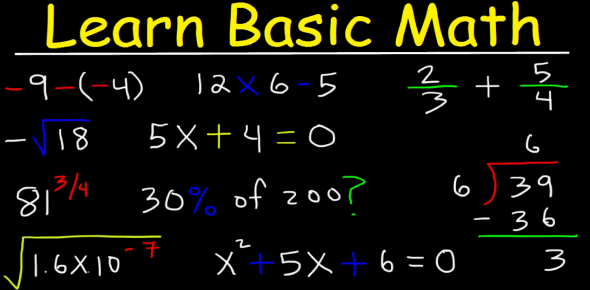# Arithmetic And Geometric Sequence

9 Questions | Attempts: 4338
ShareSettingsThis quiz is for second year high-school students. The result will show if the students learn about the lesson.

• 1.
• Find the common difference and the next term of the following sequence:
• 3, 11, 19, 27, 35,...
• A.

8

• B.

7

• C.

6

• D.

5

• E.

4

• 2.
• Find the common ratio and the seventh term of the following sequence:
• 2/9, 2/3, 2, 6, 18,...
• A.

4

• B.

3

• C.

2

• D.

1

• E.

0

• 3.
What is the 6th term of the sequence in number 3?
• A.

160

• B.

159

• C.

162

• D.

163

• E.

164

• 4.
• Find the tenth term and the n-th term of the following sequence:
• 1/2, 1, 2, 4, 8,...
• A.

156

• B.

265

• C.

625

• D.

256

• E.

526

• 5.
• Find the n-th term and the first three terms of the arithmetic sequence having a4 = 93 and a8 = 65.
• A.

411

• B.

141

• C.

114

• D.

441

• E.

144

• 6.
• Find the n-th and the 26th terms of the geometric sequence with a5 = 5/4 and a12 = 160.
• A.

2 621 440

• B.

2 621 441

• C.

2 622 444

• D.

2 623 440

• E.

2 620 442

• 7.
• Find the sum of 1 + 5 + 9 + ... + 49 + 53.
• 8.
• Find an if S4 = 26/27 and r = 1/3.
• 9.
Differentiate arithmetic and geometric sequence.
• ## Related TopicsBack to top
×

Wait!
Here's an interesting quiz for you.